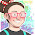## Friday, 5 February 2021

### Unit 6 - Direct Proportion

Hello, hello!
In this unit we're learning about ratios and proportions. Thus, we'll be learning today about what we call "direct proportion".Let's remember first what ratios are. A ratio is a relation between two amounts.
Then, if we have a ratio and we make one of the amounts increase/decrease, the other will do it the same way.

Here you have more examples of problems that we can solve through Direct Proportion:

Easy, right?

Here you have some games to practice direct proportions... Have fun!

I hope you find these useful!
Bye, bye, butterflies!

1.Hi Remei im studying

Martina M

2.Hello Remei. What i have to do??? Word problems or copy all the things?

David =) =) =)

1.Hello, David!
You have to copy the word problems and try to solve them. If you don't, copy the answers from the pictures.
Good job!